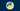# Python string to datetime and datetime to stringShare

In this article, we will learn how to convert string to date and date to string in Python with datetime. In the next sections, we will see how to use the `strftime` and `strptime` methods from the `datetime` class to format and parse dates, followed by code examples:

## Datetime to String

To convert a date or datetime object into a string we can use the `datetime` class in Python. We can format and transform a `datetime` into a string using the `strftime` method. For example, in the following snippet we use `strftime` to convert the `current_datetime` variable into multiple formats:

``````from datetime import datetime

current_datetime = datetime(2021, 3, 28, 11, 30, 0, 0)

date_time = current_datetime.strftime("%d/%m/%Y, %H:%M:%S")
print("datetime: %s" % date_time)

date = current_datetime.strftime("%d/%m/%Y")
print("date: %s" % time)

time = current_datetime.strftime("%H:%M:%S")
print("time: %s" % time)

day = current_datetime.strftime("%d")
print("day: %s" % day)

month = current_datetime.strftime("%m")
print("month: %s" % month)

year = current_datetime.strftime("%Y")
print("year: %s" % year)``````

In the example below, we used the `strftime` method to convert the `current_datetime` variable into a datetime, date, time, day, month and year format. If we run these lines of code, the output is:

``````datetime: 28/03/2021, 11:30:00
date: 28/03/2021
time: 11:30:00
day: 28
month: 03
year: 2020``````

To learn more about all the supported formats, take a look at the list of the format codes that the 1989 C standard requires:

## String to Datetime

To convert a string into a date and a datetime we can use the `datetime` class. We can parse a string into a `datetime` using the `strptime` method. In the following example, we use `strptime` to parse multiple strings in a different format and convert them into dates, time and datetime:

``````from datetime import datetime

date_time = datetime.strptime("28/03/21 11:30:00", "%d/%m/%y %H:%M:%S")
print("datetime: %s" % date_time)

time = datetime.strptime("11:30:00", "%H:%M:%S")
print("time: %s" % time)

date = datetime.strptime("28/03/21", "%d/%m/%y")
print("date: %s" % date)``````

Running the snippet, the output is:

``````datetime: 2021-03-28 11:30:00
time: 1900-01-01 11:30:00
date: 2021-03-28 00:00:00``````
If you like our post, please share it:

Polynique © 2020 - 2021, made with ❤️ by a developer, for developers.

Latest update:

All trademarks, service marks and company names are the property of their respective owners.